• 数字图像处理实例 由浅到深 包括matlab图像空间转换 数字车牌分割提取等 每个示例都有txt说明 数字图像处理实例 由浅到深 包括matlab图像空间转换 数字车牌分割提取等 每个示例都有txt说明数字图像处理
• 第1篇为MATLAB及图像基础，涵盖的内容有图像基础、MATLAB基础和MATLAB数字图像处理基础；第2篇为基于MATLAB的常见图像处理技术，涵盖的内容有数字图像的运算、数字图像增强技术、数字图像复原技术、图像分割技术、...
• 第一篇为Matlab及图像基础，第二篇为基于Matlab的常见图像处理技术，涵盖内容有数字图像的运算、数字图像增强系数、数字图像复原技术、图像分割技术、图像变换技术和色彩图像处理；第三篇为基于Matlab的高级图像处理...
• 本书系统论述了数字图像处理的基本概念、工作原理及在工程应用中的算法，精选了科学和工程中常用的多个算法，全部采用MATLAB语言编程实现，并结合实例对算法程序进行了验证和分析，其中详细讲解了图像及其分类、图像...
• 第1篇为MATLAB及图像基础，涵盖的内容有图像基础、MATLAB基础和MATLAB数字图像处理基础；第2篇为基于MATLAB的常见图像处理技术，涵盖的内容有数字图像的运算、数字图像增强技术、数字图像复原技术、图像分割技术、...
• matlab数字信号处理实例-matlab数字信号处理实例.pdf matlab数字信号处理实例matlab
• 第一篇为Matlab及图像基础，第二篇为基于Matlab的常见图像处理技术，涵盖内容有数字图像的运算、数字图像增强系数、数字图像复原技术、图像分割技术、图像变换技术和色彩图像处理；第三篇为基于Matlab的高级图像处理...
• 论文Matlab数字图像处理中的应用-MATLAB数字...针对数字图像处理中程序编写复杂且调试过程繁琐易出错的问题，介绍了一种可用于图像处理的语言——Matlab语言，并通过实例讨论了Matlab在图像处理和研究中的应用．matlab
• 本书系统论述了数字图像处理的基本概念、工作原理及在工程应用中的算法，精选了科学和工程中常用的多个算法，全部采用MATLAB语言编程实现，并结合实例对算法程序进行了验证和分析。其中，详细讲解了图像及其分类、...
• Matlab数字图像处理系统GUI实例设计（全）

千次阅读 多人点赞 2021-03-13 01:12:13
数字图像处理系统设计为例，介绍GUI系统的设计流程，同时提供两者的设计经验，如需详细了解，请下载相关资源。 数字图像处理系统 该系统基本涵盖了所有需要实现的图像处理功能： 灰度化变换、剪切、加噪和模糊...

导读

以数字图像处理系统设计为例，介绍GUI系统的设计流程，同时提供两者的设计经验，如需详细了解，请下载相关资源，资源中有完整的代码和详细的实验设计报告。

数字图像处理系统

该系统基本涵盖了所有需要实现的图像处理功能：

1. 灰度化变换、剪切、加噪和模糊处理；
2. 空域点处理中的图像反转、线性变换、对数变换、幂函数变换、均衡化增强；
3. 空域滤波中的均值滤波、中值滤波、加权滤波、Robert算子、Sobel算子、Laplace算子；
4. 频域滤波中的低通滤波和高通滤波；
5. 傅里叶变换中正变换和逆变换；
6. 人工选择阈值分割和自动阈值分割；
7. 形态学运算。

其整体框架如图所示：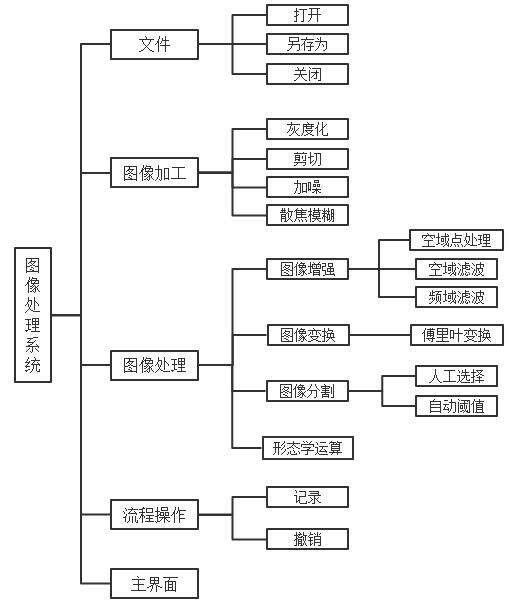其所有功能细节以及相关的代码模块都在相关资源文档中。

GUI实例设计

主界面设计：

为追求用户体验，界面设计要求简洁和布局清晰。于是便有了如下的主界面：只有两个显示窗口（“处理对象”显示窗口和“处理结果”显示窗口）和一个按钮（将“处理结果”设置为“处理对象”）

有5个功能区

1. 文件：打开（图像打开）、另存为（图像储存）、关闭（关闭系统）；
2. 图像加工：灰度化、截取、加噪、散焦模糊；
3. 图像处理：图像增强（空域点处理、空域滤波、频域滤波）、图像变换（傅里叶变换）、图像分割（人工选择分割、自动阈值分割）、形态学运算。
4. 流程操作：记录（入栈式保存“处理对象”记录）、撤销（出栈式退回上一步“记录”）；
5. 主界面：返回到主界面（两个显示窗口和一个按钮）。
 文件 图像处理 图像加工 流程操作 主面板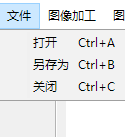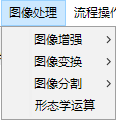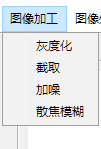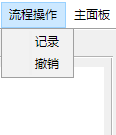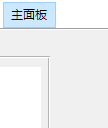控制面板设置：

当点击相关的图像功能需要用户选择时，则会出现对应的控制面板，让用户根据自己的需求设置相关参数。控制面板的实质是与用户交互。

主要控件：

此次系统，设计主要用到GUI界面设计中的各种控件功能，比如按钮、坐标轴、单选按钮、滑动条、弹出式菜单、面板等。这些控件可大致分为两种，一种是主动式控件（如“可编辑文本”、“按钮”等）、另一种是被动式控件（如“坐标轴”、“静态文本”、“面板”等）。主动式控件可放入功能响应函数，被动式控件可以显示内容。

在控件函数编辑中，有下列常用函数：

1.设置控件属性值：set(handles.控件的Tag, 控件的属性（比如’position’、’String’）, 属性值);

2.调用坐标轴作为当前显示面板：axes(handles.axes1)；axes1为坐标轴的Tag属性。

3.获取控件的数值Value或String：str2num(get(handles.edit1,’String’))或get(handles.slider1,’Value’)；edit1和slider1都是控件的Tag属性。

各个板块细节

1.文件板块：打开相应路径下的图片（图片存放路径与代码存放路径不同），然后返回当前代码路径；储存图片等。

2.图像加工模块、图像处理模块：各个函数之间的调用，各个面板控件的显示与关闭控制

该系统集成于同一个gui系统中，其中各个面板的控件分布和显示控制极具吸引力，所有详细细节在相关资源之中。

总结

以下材料详细阐述了基于MATLAB的数字图像处理系统的设计与实现问题，代码中有注释，以及有一份完整的实验报告，为图像处理的初学者和处理算法的研究人员提供一个算法演示及模拟开发的 GUI 平台，供大家学习并研究图像处理的技术方法。

请先点赞收藏再下载。

提取码: 7wak

展开全文图像处理 gui matlab
• 基于MATLAB-GUI图形界面的数字图像处理软件 本系统设计基于GUI图形界面，用matlab语言编写代码，实现功能包括图象的读取、存储、显示、直方图均衡化、阈值化、小波分解、小波重构、加噪、去噪、平滑、锐化、边缘检测...
• demo3:直方图处理（直方图---直方图） demo2:图片---图片 demo1:MATLAB自带
• 基于Matlab数字图像处理降噪方法 01 研究背景与意义 03 四种去噪方法 02 图像噪声 04 实验结果图对比 05 结论 Contents 目 录 06 源代码及函数 现实中的图像由于种种原因都是带噪声的噪声恶化了图像质量使图像模糊...
• 第1篇为MATLAB及图像基础，涵盖的内容有图像基础、MATLAB基础和MATLAB数字图像处理基础；第2篇为基于MATLAB的常见图像处理技术，涵盖的内容有数字图像的运算、数字图像增强技术、数字图像复原技术、图像分割技术、...图像处理
• MATLAB图像处理实例详解(一)

千次阅读 2019-08-17 18:06:48
常见的5种图像表示方法:分别是二进制图像,索引图像,灰度图像,RGB图像和多帧图像 1.2.1二进制图像(二值图像) 通常由一个二维数组表示,一位表示一个像素.非0即1,0表示黑色,1表示白色 1.2.2 灰度图像(单色图像) ...

1.2图像的表示方法

常见的5种图像表示方法:分别是二进制图像,索引图像,灰度图像,RGB图像和多帧图像

1.2.1二进制图像(二值图像)

通常由一个二维数组表示,一位表示一个像素.非0即1,0表示黑色,1表示白色

1.2.2 灰度图像(单色图像)

通常也由一个二维数组表示,8位表示一个像素,0表示黑色,255表示白色

1.2.3RGB图像(真彩色图像):

利用三个大小相同的二维数组表示,3个数组分别代表R G B这三个分量,每种颜色分量占8位,每一位由[0,255]中的任意值表示,那么一个像素由24位表示.

1.3 图像的数据结构

数字图像处理中常用的数据结构有矩阵、链码、拓扑结构和关系结构

矩阵用于描述图像

链码用于描述图像的边界，链码又分为四链码和八链码

拓扑结构用于描述图像的基本结构，有4-邻域，对角邻域，8-邻域。

关系结构用于描述一组目标物体之间的相互关系。

1.4计算机中图像文件的基本格式

①BMP文件格式：采用位映射存储格式，图像深度可选，从1bits-24bits，不采用任何压缩，所以占用的空间很大。

②GIF文件格式：基于LZW算法的连续色调的无损压缩格式，存储效率高，最多支持256种色彩的图像。

③JPEG文件格式：使用一种有损压缩算法，压缩比率通常在10:1到40:1，可以支持24bits真菜色。

④TIFF文件格式：适用于RGB真彩色图像。

展开全文matlab 数字图像处理
• 图像复原技术 图像在采集、传送、转换过程中会混入...在Matlab中，函数 imnoise 可以给图像加入高斯噪声、椒盐噪声、泊松分布噪声和乘性噪声 二、空域内的滤波复原方法 1、均值滤波 (1)、算术均值滤波 令 Sxy 表示...

图像复原技术

图像在采集、传送、转换过程中会混入噪声，造成图像模糊、失真、有噪声，图像复原技术的目的是使图像尽可能恢复到原本的样子。

一、常见的噪声类型

• 高斯噪声
• 椒盐噪声
• 均匀分布噪声
• 指数分布噪声
• 伽马分布噪声

在Matlab中，函数 imnoise 可以给图像加入高斯噪声、椒盐噪声、泊松分布噪声和乘性噪声

二、空域内的滤波复原方法

1、均值滤波

(1)、算术均值滤波

令 Sxy 表示中心在点(x, y)处、大小为 m * n的矩形子窗口区域(邻域)的一组坐标，然后计算这一组坐标所在像素值的平均值，将其赋值给点 (x, y)。

通俗点讲，就是选图像上的一块区域，把这块区域的所有像素点相加求平均值再赋值给这块区域中心的那个像素点，后边的几种方法只是算法不同而已。

公式为： f ^ ( x , y ) = 1 m n ∑ ( s , t ) ∈ S x y g ( s , t ) \hat{f}(x, y) = \frac{1}{mn}\sum_{(s, t) \in S_{xy}}g(s, t)

(2)、几何均值滤波

算术均值滤波是把 Sxy 所代表点的像素值求和再取平均值，而几何均值滤波是把像素值求乘再求像素的数量次幂。几何均值滤波实现的平滑可与算术均值滤波相比，且丢失的图像细节更少
公式为： f ^ ( x , y ) = [ ∏ ( s , t ) ∈ S x y g ( s , t ) ] 1 m n \hat{f}(x, y) = \left[ \prod_{(s, t) \in S_{xy}}g(s, t)\right]^{\frac{1}{mn}}

(3)、谐波均值滤波器

谐波均值滤波器对于盐粒噪声效果很好，但不适用于胡椒噪声，擅于处理高斯噪声
公式为： f ^ ( x , y ) = m n ∑ ( s , t ) ∈ S x y 1 g ( s , t ) \hat{f}(x, y) = \frac{mn}{\sum_{(s, t) \in S_{xy}} \frac{1}{g(s, t)}}

(4)、逆谐波均值滤波器

Q 称为滤波器的阶数，适用于减少或在实际中消除椒盐噪声干扰的影响。当 Q 为正时，该滤波器可消除胡椒噪声，当 Q 为负值时该滤波器可消除盐粒噪声，当 Q 为 0 时，公式可化简为算术均值滤波，当 Q = -1 时则为谐波均值滤波器。
公式为： f ^ ( x , y ) = ∑ ( s , t ) ∈ S x y g ( s , t ) Q + 1 ∑ ( s , t ) ∈ S x y g ( s , t ) Q \hat{f}(x, y) = \frac{\sum_{(s, t) \in S_{xy}}g(s, t)^{Q+1}}{\sum_{(s, t) \in S_{xy}}g(s, t)^{Q}}

下列代码为算术均值滤波和几何均值滤波的实例代码及结果：

I = im2double(I);
I = imnoise(I, 'gaussian', 0.05);   %加入高斯噪声
%算术均值滤波
PSF = fspecial('average', 3);   %创建预定义的二维滤波器
J = imfilter(I, PSF);
%几何均值滤波
K = exp(imfilter(log(I), PSF));

figure;
subplot(131);imshow(I);title('加入高斯噪声');
subplot(132);imshow(J);title('算术均值滤波');
subplot(133);imshow(K);title('几何均值滤波');

冈萨雷斯大佬的《数字图像处理》那本书上确实写了几何均值滤波比算术均值滤波要保留更多细节，我tm咋感觉是假的呢 o(一︿一+)o，结果如下：2、统计排序滤波

其实我觉得和均值滤波的原理差不多，都是选取一块区域，把这块区域里的像素们(一长串数字)进行各种运算操作。

(1)、中值滤波器

中值滤波函数：medfilt2

正如其名字一样，使用一个像素邻域中的灰度级的中值来替代该像素的值。该滤波器针对单极或双极脉冲噪声尤其有效
公式为： f ^ ( x , y ) = m e d i a n ( s , t ) ∈ S x y { g ( s , t ) } \hat{f}(x, y) = median_{(s, t) \in S_{xy}} \left\{ g(s, t) \right\}

I = im2double(I);
I = imnoise(I, 'salt & pepper', 0.05);   %加入椒盐噪声

J = medfilt2(I, [3, 3]);    %中值滤波

figure;
subplot(121);imshow(I);title('加入椒盐噪声');
subplot(122);imshow(J);title('中值滤波');

从结果可以看出，图像很好的去除了椒盐噪声，但是图像有些模糊了：(2)、最大值滤波器

就是对邻域中的所有像素点取最大值赋值给该像素点，最大值滤波器对于发现图像中的最亮点非常有用！另外它会从黑色物体边缘去除一些黑色像素
公式为： f ^ ( x , y ) = m a x ( s , t ) ∈ S x y { g ( s , t ) } \hat{f}(x, y) = max_{(s, t) \in S_{xy}} \left\{ g(s, t) \right\}

(3)、最小值滤波器

就是对邻域中的所有像素点取最小值赋值给该像素点，最小值滤波器对于发现图像中的最暗点非常有用！另外它会从白色物体边缘去除一些白色像素
公式为： f ^ ( x , y ) = m i n ( s , t ) ∈ S x y { g ( s , t ) } \hat{f}(x, y) = min_{(s, t) \in S_{xy}} \left\{ g(s, t) \right\}

(4)、中点滤波器

选取最大值与最小值的中点(就是最大最小值的平均)替换该点的像素值，结合了统计排序和求平均，对于处理随机噪声表现很好，比如高斯噪声或均匀噪声。
公式为： f ^ ( x , y ) = 1 2 [ m a x ( s , t ) ∈ S x y { g ( s , t ) } + m i n ( s , t ) ∈ S x y { g ( s , t ) } ] \hat{f}(x, y) = \frac{1}{2} [ max_{(s, t) \in S_{xy}} \left\{ g(s, t) \right\} + min_{(s, t) \in S_{xy}} \left\{ g(s, t) \right\} ]

• 二维顺序统计滤波函数：ordfilt2，可以实现中值、最大最小值滤波
I = rgb2gray(I);
I = im2double(I);
I = imnoise(I, 'salt & pepper', 0.1);   %加入椒盐噪声

domain = [1 1 1 1; 1 1 1 1; 1 1 1 1; 1 1 1 1];
J1 = ordfilt2(I, 1, domain);
J2 = ordfilt2(I, 8, domain);
J3 = ordfilt2(I, 16, domain);

figure;
subplot(221);imshow(I);title('加入椒盐噪声');
subplot(222);imshow(J1);title('最小值滤波');
subplot(223);imshow(J2);title('中值滤波');
subplot(224);imshow(J3);title('最大值滤波');

结果展示：3、自适应滤波

二维自适用去噪滤波：wiener2，自适应滤波器的性能比目前所有滤波器的性能都好

I = rgb2gray(I);

%I = imcrop(I, [100, 100, 200, 200]);    %图像裁剪

I = imnoise(I, 'gaussian', 0, 0.003);   %加入高斯噪声
[K, noise] = wiener2(I, [5, 5]);

figure;
subplot(121);imshow(I);title('加入高斯噪声');
subplot(122);imshow(K);title('自适应滤波');

可以看出，效果还不错：三、图像复原方法

1、逆滤波

大意就是我们知道了原图受到了什么算法(叫做退化函数 H )带来的噪声，那么我们从噪声图反着用这个算法就可以推算出原图。
不过即使知道了退化函数，也还是不能完全恢复到原图。

I = im2double(I);
[m, n] = size(I);
M = 2 * m;
N = 2 * n;

u = -m / 2:(m / 2 - 1);
v = -n / 2:(n / 2 - 1);
[U, V] = meshgrid(u, v);           %基于向量 u 和 v 中包含的坐标返回二维网格坐标
D = sqrt(U.^2 + V.^2);
D0 = 130;                           %滤波器截止频率为20
H = exp(-(D.^2)./(2 * (D0^2)));
N = 0.01 * ones(size(I, 1), size(I, 2));
N = imnoise(N, 'gaussian', 0, 0.001);
J = fftfilter(I, H) + N;

HC = zeros(m, n);
M1 = H > 0.1;
HC(M1) = 1 ./ H(M1);
K = fftfilter(J, HC);
HC = zeros(m, n);
M2 = H > 0.01;
HC(M2) = 1 ./ H(M2);
L = fftfilter(J, HC);

figure;
subplot(221);imshow(J);
subplot(222);imshow(J, []);
subplot(223);imshow(K, []);
subplot(224);imshow(L, []);

其中，fftfilter函数如下：

function Z = fftfilter(X, H)
F = fft2(X, size(H, 1), size(H, 2));
Z = H .* F;
Z = ifftshift(Z);
Z = abs(ifft2(Z));
Z = Z(1:size(X, 1), 1:size(X, 2));
end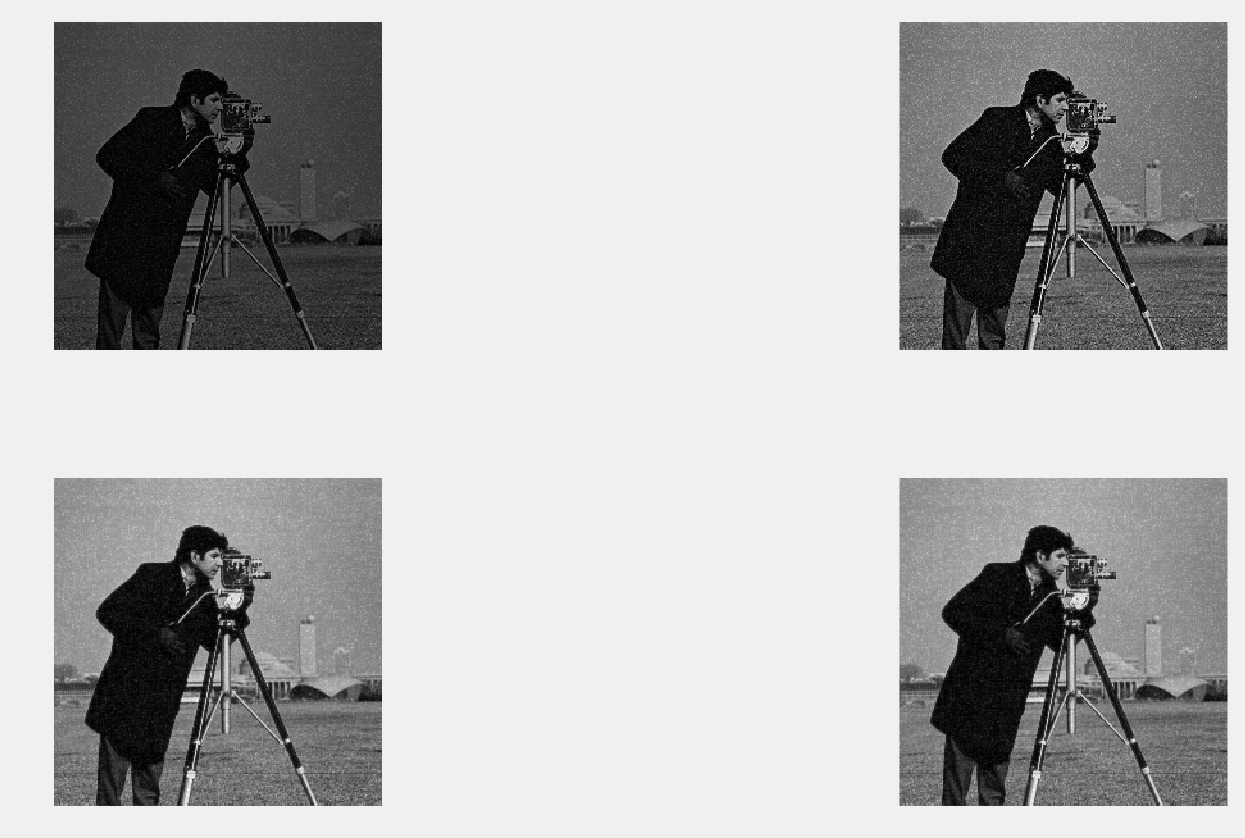完整目录

展开全文matlab 数字图像处理
• 第1篇为MATLAB及图像基础，涵盖的内容有图像基础、MATLAB基础和MATLAB数字图像处理基础；第2篇为基于MATLAB的常见图像处理技术，涵盖的内容有数字图像的运算、数字图像增强技术、数字图像复原技术、图像分割技术、...MATLAB
•matlab
•matlab
• 冈萨雷斯数字图像处理MATLAB版配套资料，包含图片和源码图片
•matlab
• 第1篇为MATLAB及图像基础，涵盖的内容有图像基础、MATLAB基础和MATLAB数字图像处理基础；第2篇为基于MATLAB的常见图像处理技术，涵盖的内容有数字图像的运算、数字图像增强技术、数字图像复原技术、图像分割技术、...
• 实例简介】现代数字图像处理技术提高及应用案例详解(Matlab版)--源代码【实例截图】【核心代码】现代数字图像处理技术提高及应用案例详解(Matlab版)└── 现代数字图像处理技术提高及应用案例详解(Matlab版)----...

【实例简介】

现代数字图像处理技术提高及应用案例详解(Matlab版)--源代码

【实例截图】

【核心代码】

现代数字图像处理技术提高及应用案例详解(Matlab版)

└── 现代数字图像处理技术提高及应用案例详解(Matlab版)----源码

├── 1.1 图像多分辨率金字塔

│   ├── ex1.m

│   ├── ex2.m

│   ├── genPyr.m

│   ├── pyr_expand.m

│   ├── pyr_reduce.m

│   ├── qingdao.JPG

│   ├── tiantan.jpg

│   └── 程序调用说明.docx

├── 1.2图像的矩特征

│   ├── 1.2 运行说明.doc

│   ├── invariable_moment.m

│   ├── qingdao.JPG

│   └── zernike.m

├── 1.3 图像的边缘检测

│   ├── 1.3 程序运行说明.doc

│   ├── canny_edge.m

│   ├── cannyFindLocalMaxima.m

│   ├── diaohua.jpg

│   ├── ex6.m

│   ├── ex7.m

│   ├── ex8.m

│   ├── ex9.m

│   ├── hehua.JPG

│   ├── log_edge.m

│   ├── qipan.JPG

│   ├── susan.m

│   ├── susan_threshold.m

│   └── xinglong.jpg

├── 1.4图像的斑点检测

│   ├── 1.4 程序使用说明.doc

│   ├── draw.m

│   ├── gilles.m

│   ├── log_Blob.m

│   ├── patrol.jpg

│   └── sunflower.jpg

├── 1.5 角点特征检测

│   ├── 1.5 程序调用说明.docx

│   ├── door.jpg

│   ├── draw.m

│   ├── ex2.m

│   ├── findLocalMaximum.m

│   ├── Harris1.m

│   ├── harrislaplace.m

│   ├── hua.jpg

│   └── long.JPG

├── 1.6 尺度不变特征提取

│   ├── 1.6节程序运行说明.doc

│   ├── display_keypoints.m

│   ├── gaussian_filter.m

│   ├── integralimage.m

│   ├── resizeImageFig.m

│   └── SIFT.m

├── 2.1图像目标边界描述

│   ├── 2.1 程序运行说明.docx

│   ├── chaincode4.m

│   ├── chaincode8.m

│   └── ex3.m

├── 2.2 图像分割技术

│   ├── 2.2-2程序调用说明.doc

│   ├── baihe.jpg

│   ├── ex1.m

│   ├── ex2.m

│   ├── ex3.m

│   ├── ex4.m

│   ├── hehua.JPG

│   ├── kmeans.m

│   ├── medtest.png

│   ├── moli.jpg

│   ├── regiongrowing.m

│   ├── shuixian.jpg

│   ├── thresh_md.m

│   ├── tshape.png

│   └── xiangrikui.jpg

├── 2.3图像配准技术

│   ├── 2.3程序运行说明.docx

│   ├── correlatiomatrix.m

│   ├── draw2.m

│   ├── ex2.m

│   ├── getRegionValue.m

│   ├── kp_harris.m

│   ├── matchbycorrelation.m

│   ├── match.m

│   ├── scence1.JPG

│   ├── scence2.JPG

│   ├── template_matching.m

│   └── test.m

├── 2.4图像融合技术

│   ├── area_var_match.m

│   ├── clock1.bmp

│   ├── clock2.bmp

│   ├── dec2.m

│   ├── downspl.m

│   ├── es2.m

│   ├── ex1.m

│   ├── ex2.m

│   ├── ex3.m

│   ├── fuse_lap.m

│   ├── lowfrefus.m

│   ├── modmat.m

│   ├── mydwt2.m

│   ├── mydwt.m

│   ├── mywavedec2.m

│   ├── selb.m

│   ├── selc.m

│   ├── submat.m

│   ├── undec2.m

│   ├── weivec.m

│   └── 程序运行说明.doc

├── 3.11指纹识别技术及其实现

│   ├── 3.11-10.txt

│   ├── 3.11-11.txt

│   ├── 3.11-12.txt

│   ├── 3.11-13.txt

│   ├── 3.11-14.txt

│   ├── 3.11-15.txt

│   ├── 3.11-6.txt

│   ├── 3.11-7.txt

│   ├── 3.11-8.txt

│   ├── 3.11-9.txt

│   ├── 例程3.11-1.txt

│   ├── 例程3.11-2.txt

│   ├── 例程3.11-3.txt

│   ├── 例程3.11-4.txt

│   └── 例程3.11-5.txt

├── 3.12 基于图像的车牌自动识别技术

│   ├── car.jpg

│   ├── ex1.m

│   ├── P.bmp

│   └── 程序运行说明.doc

├── 3.13 数字图像实时稳定技术及其实现

│   ├── getGrayCodeBitPlane.m

│   ├── imageStabilizeMain.m

│   ├── stabilizeMovie_GCBPM.m

│   └── 程序运行说明.doc

├── 3.14 基于帧间差分法的运动目标检测

│   ├── ccbr1.avi

│   ├── ex1.m

│   ├── tracking.m

│   └── 程序运行说明.doc

├── 3.15 基于光流场的运动估计

│   ├── computeDerivatives.m

│   ├── gaussFilter.m

│   ├── HS.m

│   ├── plotFlow.m

│   ├── smoothImg.m

│   ├── yos10.tif

│   ├── yos9.tif

│   └── 程序说明.doc

├── 3.18 meanshift

│   ├── color_example.m

│   ├── color_object_tracking2.m

│   ├── compute_kernelmatrix.m

│   ├── compute_k_hist.m

│   ├── compute_wi.m

│   ├── object_tracking.m

│   ├── show_target.m

│   ├── track.m

│   └── 程序运行说明.doc

├── 3.19 基于Kalman滤波的目标跟踪

│   ├── DATA

│   │   ├── 10.jpg

│   │   ├── 11.jpg

│   │   ├── 12.jpg

│   │   ├── 13.jpg

│   │   ├── 14.jpg

│   │   ├── 15.jpg

│   │   ├── 16.jpg

│   │   ├── 17.jpg

│   │   ├── 18.jpg

│   │   ├── 19.jpg

│   │   ├── 1.jpg

│   │   ├── 20.jpg

│   │   ├── 21.jpg

│   │   ├── 22.jpg

│   │   ├── 23.jpg

│   │   ├── 24.jpg

│   │   ├── 25.jpg

│   │   ├── 26.jpg

│   │   ├── 27.jpg

│   │   ├── 28.jpg

│   │   ├── 29.jpg

│   │   ├── 2.jpg

│   │   ├── 30.jpg

│   │   ├── 31.jpg

│   │   ├── 32.jpg

│   │   ├── 33.jpg

│   │   ├── 34.jpg

│   │   ├── 35.jpg

│   │   ├── 36.jpg

│   │   ├── 37.jpg

│   │   ├── 38.jpg

│   │   ├── 39.jpg

│   │   ├── 3.jpg

│   │   ├── 40.jpg

│   │   ├── 41.jpg

│   │   ├── 42.jpg

│   │   ├── 43.jpg

│   │   ├── 44.jpg

│   │   ├── 45.jpg

│   │   ├── 46.jpg

│   │   ├── 47.jpg

│   │   ├── 48.jpg

│   │   ├── 49.jpg

│   │   ├── 4.jpg

│   │   ├── 50.jpg

│   │   ├── 51.jpg

│   │   ├── 52.jpg

│   │   ├── 53.jpg

│   │   ├── 54.jpg

│   │   ├── 55.jpg

│   │   ├── 56.jpg

│   │   ├── 57.jpg

│   │   ├── 58.jpg

│   │   ├── 59.jpg

│   │   ├── 5.jpg

│   │   ├── 60.jpg

│   │   ├── 6.jpg

│   │   ├── 7.jpg

│   │   ├── 8.jpg

│   │   ├── 9.jpg

│   │   └── Thumbs.db

│   ├── ex1.m

│   ├── extractball.m

│   ├── main.m

│   └── 程序运行说明.doc

├── 3.1 图像去噪技术及其实现

│   ├── 3.1程序说明.docx

│   ├── ex1.m

│   ├── ex2.m

│   ├── life.jpg

│   └── wangshi.jpg

├── 3.20 基于Hough变换的人眼虹膜定位方法

│   ├── ex1.m

│   ├── yanjing.bmp

│   └── 程序运行说明.doc

├── 3.21 基于模糊集的图像增强方法

│   ├── ex1.m

│   ├── lena.JPG

│   └── 程序运行说明.doc

├── 3.22 基于KL变换的人脸识别技术

│   ├── ex1.m

│   ├── ScaleImage.m

│   └── 程序运行说明.doc

├── 3.24 基于蚁群算法的图像边缘检测

│   ├── ant128_edge_aco_1.jpg

│   ├── ant128_edge_aco_2.jpg

│   ├── ant.jpg

│   ├── edge_ACO.m

│   └── 程序运行说明.doc

├── 3.25 基于脉冲耦合神经网络的图像分割

│   ├── lena.JPG

│   ├── pcnn.m

│   └── 程序运行说明.doc

├── 3.2畸变校正技术

│   ├── gmodify.m

│   └── 按如下说明调用.doc

├── 3.3图像拼接技术

│   ├── appendimages.m

│   ├── findHomography.m

│   ├── hall1.JPG

│   ├── hall2.JPG

│   ├── imMosaic.m

│   ├── mosaic_a.jpg

│   ├── mosaic_hall.jpg

│   ├── mosaicTest.m

│   ├── randIndex.m

│   ├── ransac1.m

│   ├── sift.m

│   ├── siftMatch.m

│   ├── siftWin32.exe

│   ├── solveHomo.m

│   ├── tmp.key

│   └── 程序运行说明.doc

├── 3.4 数字水印技术及其实现

│   ├── ex1.m

│   ├── ex2.m

│   ├── lena.bmp

│   ├── lww.avi

│   ├── mark.bmp

│   ├── withed11.avi

│   ├── Y.BMP

│   └── 程序使用说明.doc

├── 3.5 图像压缩技术及其实现

│   ├── ex1.m

│   ├── ex2.m

│   ├── ex3.m

│   ├── hangtian.jpg

│   ├── hundungen.m

│   ├── robot.jpg

│   ├── tank.JPG

│   └── 运行说明.doc

├── 3.6改进型数字图像中直线特征的快速检测方法

│   ├── myhough.m

│   └── 程序运行说明.doc

├── 3.7 基于最大类间方差阈值与遗传算法的道路分割

│   ├── main.m

│   └── 程序运行说明：.doc

├── 3.8 数字图像处理在医疗领域的应用

│   ├── ex1.m

│   ├── intrans.m

│   ├── ranseti.bmp

│   └── 程序运行说明.doc

├── 3.9 基于红外图像的弱小目标检测与跟踪

│   ├── main.m

│   ├── target_detect.m

│   ├── target_refine.m

│   └── 程序运行说明.doc

├── 4.2 基于GUI交互操作的数字图像处理实现.rar

└── 4.3 在VC 环境下调用MATLAB实现数字图像处理.rar

33 directories, 285 files

展开全文• 目 录章 初识数字图像处理与识别 11.1 数字图像 11.1.1 什么是数字图像 11.1.2 数字图像的显示 11.1.3 数字图像的分类 21.1.4 数字图像的实质 ...61.2.1 从图像处理到图像识别 61.2.2 数字图像处理与识别的应用实例 ...
• MATLAB图像处理的实例

千次阅读 2020-11-20 17:02:53
MATLAB实现的图像处理基础的案例，如HSI,直方图统计等。算法 图像处理 matlab 计算机视觉
• 一、利用MATLAB生成LOG算子图像 %%用matlab生成LOG图像 x=-2:0.06:2 y=-2:0.06:2 sigma=0.6 y=y'; for i=1:(4/0.06+1) xx(i,:)=x; yy(:,i)=y; end r=1/(2*pi*sigma^4)*((xx.^2+yy.^2)/(sigma^2)-2).*exp(-(xx.^2+...matlab 算法 矩阵
• MATLAB数字图像水印摘要：介绍了数字水印的原理和应用方法，研究了基于离散余弦变换(DCT)的数字图像水印算法，并借助MATLAB编S-Y-具，实现数字水印的嵌入、提取和攻击测试。实验结果表明，对于通常的压缩编码，该...算法 计算机视觉 人工智能 编程语言 python
• 论文Matlab数字图像处理中的应用-Matlab数字...针对数字图像处理中程序编写复杂且调试过程繁琐易出错的问题，介绍了一种可用于图像处理的语言——Matlab语言，并通过实例讨论了Matlab在图像处理和研究中的应用．matlab...

matlab数字图像实例matlab 订阅